Search by Topic

Resources tagged with Biology similar to PDF:

Filter by: Content type:
Age range:
Challenge level:

There are 63 results

Broad Topics > Applications > BiologyStats Statements

Age 16 to 18 Challenge Level:

Are these statistical statements sometimes, always or never true? Or it is impossible to say?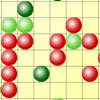Epidemic Modelling

Age 14 to 18 Challenge Level:

Use the computer to model an epidemic. Try out public health policies to control the spread of the epidemic, to minimise the number of sick days and deaths.Robot Camera

Age 14 to 16 Challenge Level:

Could nanotechnology be used to see if an artery is blocked? Or is this just science fiction?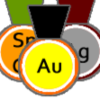Alternative Record Book

Age 14 to 18 Challenge Level:

In which Olympic event does a human travel fastest? Decide which events to include in your Alternative Record Book.Population Dynamics - Part 6

Age 16 to 18 Challenge Level:

Sixth in our series of problems on population dynamics for advanced students.Population Ecology Using Probability

Age 16 to 18 Challenge Level:

An advanced mathematical exploration supporting our series of articles on population dynamics for advanced students.Age 16 to 18 Challenge Level:

Use combinatoric probabilities to work out the probability that you are genetically unique!Genetic Intrigue

Age 16 to 18

Dip your toe into the fascinating topic of genetics. From Mendel's theories to some cutting edge experimental techniques, this article gives an insight into some of the processes underlying. . . .Predator - Prey Systems

Age 16 to 18 Challenge Level:

See how differential equations might be used to make a realistic model of a system containing predators and their prey.Why Multiply When You're about to Divide?

Age 16 to 18 Challenge Level:

A brief introduction to PCR and restriction mapping, with relevant calculations...Population Dynamics Collection

Age 16 to 18 Challenge Level:

This is our collection of tasks on the mathematical theme of 'Population Dynamics' for advanced students and those interested in mathematical modelling.Population Dynamics - Part 5

Age 16 to 18 Challenge Level:

Fifth in our series of problems on population dynamics for advanced students.Light Absorbance

Age 16 to 18 Challenge Level:

Test your skills at this light-absorbance calculation.Population Dynamics - Part 4

Age 16 to 18 Challenge Level:

Fourth in our series of problems on population dynamics for advanced students.Ratios and Dilutions

Age 14 to 16 Challenge Level:

Scientists often require solutions which are diluted to a particular concentration. In this problem, you can explore the mathematics of simple dilutionsWhose Line Graph Is it Anyway?

Age 16 to 18 Challenge Level:

Which line graph, equations and physical processes go together?Population Dynamics

Age 16 to 18 Challenge Level:

This problem opens a major sequence of activities on the mathematics of population dynamics for advanced students.Branching Processes and Extinction

Age 16 to 18 Challenge Level:

An advanced mathematical exploration supporting our series of articles on population dynamics for advanced students.Population Dynamics - Part 1

Age 16 to 18 Challenge Level:

First in our series of problems on population dynamics for advanced students.Population Dynamics - Part 2

Age 16 to 18 Challenge Level:

Second in our series of problems on population dynamics for advanced students.Population Dynamics - Part 3

Age 16 to 18 Challenge Level:

Third in our series of problems on population dynamics for advanced students.Concentrations

Age 16 to 18 Challenge Level:

Use the interactivity to practise your skills with concentrations and molarity.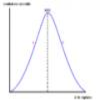Graphic Biology

Age 16 to 18 Challenge Level:

Several graphs of the sort occurring commonly in biology are given. How many processes can you map to each graph?Approximately Certain

Age 14 to 18 Challenge Level:

Estimate these curious quantities sufficiently accurately that you can rank them in order of sizeExtreme Dissociation

Age 16 to 18 Challenge Level:

In this question we push the pH formula to its theoretical limits.Bionrich

Age 14 to 18 Challenge Level:

bioNRICH is the area of the stemNRICH site devoted to the mathematics underlying the study of the biological sciences, designed to help develop the mathematics required to get the most from your. . . .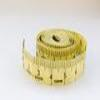Bigger or Smaller?

Age 14 to 16 Challenge Level:

When you change the units, do the numbers get bigger or smaller?Mathematical Issues for Biologists

Age 16 to 18 Challenge Level:

STEM students at university often encounter mathematical difficulties. This articles highlights the 8 key problems for biologists.Packing 3D Shapes

Age 14 to 16 Challenge Level:

What 3D shapes occur in nature. How efficiently can you pack these shapes together?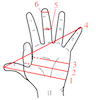Age 14 to 16 Challenge Level:

Which units would you choose best to fit these situations?Investigating the Dilution Series

Age 14 to 16 Challenge Level:

Which dilutions can you make using only 10ml pipettes?Mixing Ph

Age 16 to 18 Challenge Level:

Use the logarithm to work out these pH values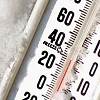Ph Temperature

Age 16 to 18 Challenge Level:

At what temperature is the pH of water exactly 7?Real-life Equations

Age 16 to 18 Challenge Level:

Here are several equations from real life. Can you work out which measurements are possible from each equation?Blood Buffers

Age 16 to 18 Challenge Level:

Investigate the mathematics behind blood buffers and derive the form of a titration curve.A Question of Scale

Age 14 to 16 Challenge Level:

Use your skill and knowledge to place various scientific lengths in order of size. Can you judge the length of objects with sizes ranging from 1 Angstrom to 1 million km with no wrong attempts?Chi-squared Faker

Age 16 to 18 Challenge Level:

How would you massage the data in this Chi-squared test to both accept and reject the hypothesis?The Genes of Gilgamesh

Age 14 to 16 Challenge Level:

Can you work out the parentage of the ancient hero Gilgamesh?Very Old Man

Age 16 to 18 Challenge Level:

Is the age of this very old man statistically believable?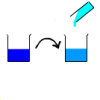Dilution Series Calculator

Age 14 to 16 Challenge Level:

Which dilutions can you make using 10ml pipettes and 100ml measuring cylinders?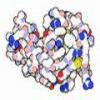Mixed up Mixture

Age 14 to 16 Challenge Level:

Can you fill in the mixed up numbers in this dilution calculation?Fitting Flat Shapes

Age 16 to 18 Challenge Level:

How efficiently can various flat shapes be fitted together?Core Scientific Mathematics

Age 14 to 18 Challenge Level:

This is the area of the advanced stemNRICH site devoted to the core applied mathematics underlying the sciences.Catalyse That!

Age 16 to 18 Challenge Level:

Can you work out how to produce the right amount of chemical in a temperature-dependent reaction?Bird-brained

Age 16 to 18 Challenge Level:

How many eggs should a bird lay to maximise the number of chicks that will hatch? An introduction to optimisation.Exact Dilutions

Age 14 to 16 Challenge Level:

Which exact dilution ratios can you make using only 2 dilutions?Chemnrich

Age 14 to 18 Challenge Level:

chemNRICH is the area of the stemNRICH site devoted to the mathematics underlying the study of chemistry, designed to help develop the mathematics required to get the most from your study. . . .Drug Stabiliser

Age 16 to 18 Challenge Level:

How does the half-life of a drug affect the build up of medication in the body over time?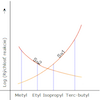Reaction Types

Age 16 to 18 Challenge Level:

Explore the rates of growth of the sorts of simple polynomials often used in mathematical modelling.Neural Nets

Age 16 to 18

Find out some of the mathematics behind neural networks.